# HSSlive: Plus One & Plus Two Notes & Solutions for Kerala State Board

## BSEB Class 12 Chemistry The d-and f-Block Elements Textbook Solutions PDF: Download Bihar Board STD 12th Chemistry The d-and f-Block Elements Book AnswersBSEB Class 12 Chemistry The d-and f-Block Elements Textbook Solutions PDF: Download Bihar Board STD 12th Chemistry The d-and f-Block Elements Book Answers

BSEB Class 12th Chemistry The d-and f-Block Elements Textbooks Solutions and answers for students are now available in pdf format. Bihar Board Class 12th Chemistry The d-and f-Block Elements Book answers and solutions are one of the most important study materials for any student. The Bihar Board Class 12th Chemistry The d-and f-Block Elements books are published by the Bihar Board Publishers. These Bihar Board Class 12th Chemistry The d-and f-Block Elements textbooks are prepared by a group of expert faculty members. Students can download these BSEB STD 12th Chemistry The d-and f-Block Elements book solutions pdf online from this page.

## Bihar Board Class 12th Chemistry The d-and f-Block Elements Books Solutions

 Board BSEB Materials Textbook Solutions/Guide Format DOC/PDF Class 12th Subject Chemistry The d-and f-Block Elements Chapters All Provider Hsslive

## How to download Bihar Board Class 12th Chemistry The d-and f-Block Elements Textbook Solutions Answers PDF Online?

2. Click on the Bihar Board Class 12th Chemistry The d-and f-Block Elements Answers.
3. Look for your Bihar Board STD 12th Chemistry The d-and f-Block Elements Textbooks PDF.
4. Now download or read the Bihar Board Class 12th Chemistry The d-and f-Block Elements Textbook Solutions for PDF Free.

Find below the list of all BSEB Class 12th Chemistry The d-and f-Block Elements Textbook Solutions for PDF’s for you to download and prepare for the upcoming exams:

### Bihar Board Class 12 Chemistry The d-and f-Block Elements Intext Questions and Answers

Question 1.
Silver atom has completely filled d orbitals (4d10) in its ground state. How can you say that it is a transition element?
The silver atom has incomplete d-orbital (4d9) in its oxidation state of IF. Hence it is treated as a transition element.

Question 2.
In the series Sc (Z = 21) to Zn (Z = 30), the enthalpy of atomisation of zinc is the lowest, i.e., 126 kj mol-1. Why?
Zinc has the lowest enthalpy of atomisation because of its electronic configuration of 3d104s2, atoms of zinc have weak interatomic interaction hence weak bonding between atoms resulting in lower the enthalpy of atomisation. In all other metals of the 3d series electrons from 3d orbitals are always involved in the formation of metallic bonds.

Question 3.
Which of the 3d series of the transition metals exihibits the largest number of oxidation states and why?
Mn (Z = 25) shows the maximum number of oxidation states because it utilizes all the seven valence electrons (3d54s2) for bond formations in different compounds. In compounds like MnCl2, Mn2O3, MnO2 K2MnO4, KMnO4, manganese shows oxidation states of + 2, + 3, + 4, + 6, + 7 respectively.

Question 4.
The Eθ (M2+/M) value for copper is positive (+ 0.34V). What is possibly the reason for this?
[Hint: Consider its high ΔaHθ and low ΔhydHθ]
The Eθ for Cu++/Cu is + 0.34. Electrode potential values depend upon factors such as (i) energy of sublimation of the metal, (ii) the ionisation energy and (iii) hydration energy. The high energy required to transform Cu (s) to Cu2+ (aq) is not balanced by the low value of its hydration energy. Hence it shows a positive value of its reduction potential.

Question 5.
How would you account for the irregular variation of ionisation enthalpies (first and second) in the first series of the transition elements?
Depending upon the electronic configuration of 3d1- 10 4s1-2 of first transition series and the number of electrons used for bond formation, the first and 2nd ionisation enthalpies vary in an irregular manner. This irregular trend can be accounted for by considering that the removal of one electron changes the relative energies of 4s and 3d orbitals so that the unipositive ions have dn configuration with no 4s electrons. There is thus a reorganisation of energy accompanying ionisation with some gains in exchange energy as the number of electrons increases and from the transference of s-electrons into d orbitals.

There is the generally expected increasing trend in the values as the effective nuclear charge increases with the value of Cr being lower because of the absence of any change in the d configuration and tlie value for Zn being relatively high because it represents an ionization from the 4s level. To form M2+ ions from the gaseous atoms, the sum of the first and 2nd ionisation enthalpies is required in addition to the enthalpy of atomisation for each element.

The dominat term is the 2nd IE which shows unusually high values for Cr and Cu where the d5 and d10 configurations of the M+ ions are disrupted with considerable loss of exchange energy. The value for Zn is correspondingly low as the ionisation consists of the removal of an electron from 4s1 which allows the formation of the stable d10 configuration.

Question 6.
Why is the highest oxidation state of a metal exhibited in its oxides or fluoride only?
The highest oxidation state of a metal is exhibited in oxides or fluorides only because of their small size and high electro negativity, both of them can oxidise the metal to its highest oxidation state:

Question 7.
Which is stronger reducing agent Cr2+ or Fe2+ and why?
The reduction potentials for Cr2+ and Fe2+are as given:
Cr3+ + e → Cr2+; E° = -0.41V
Fe3+ + e→ Fe2+; E° = +0.77 V
or Cr2+ → Cr3+ + e; E°OX = + 0.41 V
Fe2+ → Fe3+ + e; E°OX = – 0.77 V
On the basis of oxidation potentials above Cr2+ is more likely to get oxidized to Cr3+ than Fe2+ which has a negative oxidation potential. Hence Cr2+ is more reducing in nature than Fe2+. [3rd I.E. required to convert Fe2+ (3d6) to Fe3+ (3d5 – stable electronic configuration) is very small]. In a medium (like water) d3 is more stable as compared to d5.

Question 8.
Calculate the ‘spin only’ magnetic moment of M2+ (aq) ion (Z = 27).
With atomic number 27, the divalent ion in aqueous solution will have d7 configuration (three unpaired electrons)
M = 27 = 1s2, 2s22p6, 3s23p63d7 4s2
M2+ = 1s2,2s22p6, 3s23p6, 3d7.
The magnetic moment µ is (spin only)
µ = 3(3+2)‾‾‾‾‾‾‾‾√ [∵ µ = 𝑛(𝑛+2)‾‾‾‾‾‾‾‾√]
= 15‾‾‾√ B.M. =3.87B.M.

Question 9.
Explain why Cu+ ion is not stable in aqueous solutions?
The oxidation state of a metal in a solvent depends upon the nature of the solvent. The metal in a particular oxidation state may undergo oxidation or reduction in the solvent under appropriate conditions. For example, Cu+ is unstable in water as it undergoes oxidation to Cu2+ and reduction to Cu, i.e.; it undergoes disproportionation
2 Cu+ (aq) → Cu2+ (aq) + Cu (s)
The E° value for this reaction is favourable.

Question 10.
Actinoid contraction is greater from element to element than lanthanoid contraction. Why?
Though the actinoid contraction is like lanthanoid contraction, this contraction is greater from element to element resulting from poor shielding by 5f electrons as compared to 4f electrons of lanthanoids.

### Bihar Board Class 12 Chemistry The d-and f-Block Elements Text Book Questions and Answers

Question 1.
Write down the electronic configuration of
(a) Cr3+,
(b) Pm3+,
(c) Cu+,
(d) Ce4+,
(e) CO2+,
(f) Lu2+,
(g) Mn2+,
(h) Th4+.
(a) Cr3+ = [Ar]18 3d3
(b) Pm3+= [Xe]544f5
(c) Cu+ = [Ar]18 3d10
(d) Ce4+ = [Xe]54
(e) CO2+ = [Ar]18 3d7
(f) Lu2+ = [Xe]54 4f14 5d1
(g) Mn2+= [Ar]183d5
(h) Th4+ = [Rn]86

Question 2.
Why are Mn2+ compounds more stable than Fe2+ towards oxidation to their+ 3 state?
Mn2+= [Ar]3d5
Fe2+ [Ar]3d6
Mn2+ has exactly half-filled electronic configuration in its 3d- subshell which is extremely stable. Therefore, 3rd ionisation enthalpy is very high, i.e., 3rd e cannot be lost easily.
∴ Mn2+ has no tendency to lose an extra electron to undergo oxidation as compared to Fe2+ which has one electron extra (than the exactly half-filled configuration) which it can lose readily to undergo oxidation to Fe3+.
Fe2+ → Fe3+ + e.

Question 3.
Explain briefly how + 2 state becomes more and more stable in the first half of the first-row transition elements with increasing atomic number.
The Sum IE1 + IE2 increases. As a result, the standard reduction potentials (E°) becomes less and less negative. Hence, the tendency to form M2+ ion decreases. The greater stability of + 2 state for Mn is due to half-filled d-subshell (3d5). So, the first member of the first transition series is having electronic configuration of SdHs2. It will prefer to lose all the three electrons to form Argon core. Therefore, it prefers to shew an oxidation state of + 3 more than + 2.

Question 4.
To what extent do the electronic configurations decide the stability of oxidation states in the first series of the transition elements? Illustrate your answer with examples.
In the first transition series, the oxidation states which lead to 3d° (empty 3d orbital), 3d5 (exactly half-filled) or 3d10 (completely filled) electronic arrangements are more stable. Thus Sc (III) with 3d° is more stable than Sc (II) with 3d1.
Electronic configuration of Mn (Z = 25) is-[Ar] 3d54s2. It shows oxidation states from + 2 to + 7, but Mn (II) is most stable because of its configuration [Ar] 3d5.

Question 5.
What may be the stable oxidation state of the transition element with the following d electron configurations in the ground state of their atoms: 3d3,3d5,3d8 and 3d4?
The most stable oxidation state for 3d3 4s2 is + 5. (3d°) + 3 and + 4
The most stable ox. state for 3d5 4s1 = + 6 (3d°) and also 1+3 and + 4.
The most stable ox. state for 3d5 4s2 = + 2, + 7 (3d5, 3d°) and also + 4 and + 6.
The most stable ox. state for 3d8 4s2 = + 2
The most stable ox. state for 3d4. There is no d4 configuration in ground state.
The maximum oxidation states of reasonable stability correspond to the sum of s and d-electrons up to Mn. After that, there is abrupt decrease in the stability of higher oxidation states.

Question 6.
Name the ox metal anions of the first series of the transition metals in which the metal exhibits the oxidation state equal to its group number.

• Dichromate ion (Cr2O72-) and chromate ion (Cr2O42-) in oxidation state of Cr is VI and its group No. is also 6.
• MnO4: Permanganate ion. Here ox. state of Mn is VII and its group no. is 7.
• Vandate: VO3; Here ox. no. of V = + 5 = group No. 5

Question 7.
What is lanthanoid contraction? What are the consequence of lanthanoid contraction?
Lanthanoid or Lanthanide Contraction-The Lanthanoids have electronic configuration with 6s2 common to all with variable occupancy of 4f level. But all try positive ions (the most stable oxidation state of all the lanthanoids) are of the form 4fn (n = 1 to n = 14. With increasing atomic number) we see a regular decrease in their ionic y radii from 106 pm in La3+ to 85 pm in Lu3+. This regular decrease of average 1.5 pm per ion from La3+ to Lu3+ is termed LANTHANOID. CONTRACTION. It is attributed to the IMPERFECT SHIELDING of one f-electron by another f-electron in the same shell because of the highly diffused shape of f-orbitals.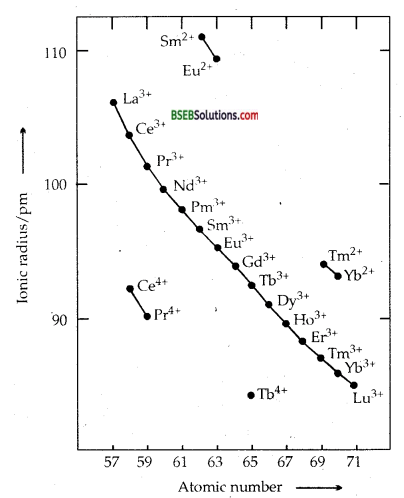CONSEQUENCES OF LANTHANOID CONTRACTION
1. The Lanthanoid contraction, which is a unique feature of the chemistry of lanthanoids has far-reaching consequences in the chemistry of the third transition series of the elements. It causes the radii of the members of the III transition series to be very similar to these of the corresponding members of the second series.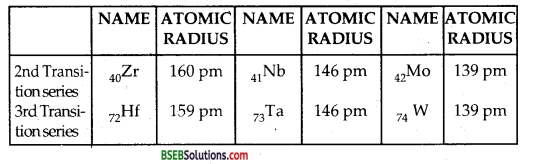Due to similar sizes; chemistry of Zr and Hf; Nb and Ta; Mo and W is similar. They show close resemblance in their properties.
2. There is a small increase in the electronegativities of the trivalent ion.
3. There is a small increase in E° (standard electrode potential) for the reduction.
M3+ + 3e → M (g)
4. There is a decrease in basic strength of oxides and hydroxides along the series.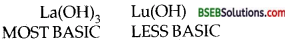5. On account of Lanthanoid contraction/there is a slight variation in chemical properties of the lanthanides which is made use of in the separation of individual lanthanides by ION-EXCHANGE methods.

Question 8.
What are the characteristics of the transition elements and why are they called transition elements? Which of the d-block elements may not be regarded as the transition elements?
The d-block elements are called TRANSITION ELEMENTS because these elements represent change (or transition) in properties from most positive electropositive s-block elements to least electropositive p-block elements. They have sandwiched betweens- block of the periodic table (highly reactive metals) and p-block (mostly non-metals). The last electron enters penultimately [(n – 1) d] subshells in the atoms of these elements.

These elements have partially filled d- subshells in their elementary states or in their commonly occurring oxidation states. Zn, Cd, Hg do not possess partially filled inner, d- orbitals either in their elementary states or in their common oxidation states.
Zn = 30 = [ Ar] 3d10 4s2 Zn (II) = 3d10
Cd = 48 = [Kr] 4d10 5s2 Cd (II) = 4d10
Hg = 80 = [Xe] 4f145d106s2 Hg (II) = 5d10

∴ Zn, Cd, Hg (belonging to 12th group of the periodic table) may notbe regarded as transition elements. However, they have been treated along with other transition elements because they show similarities in some of their properties like complex formation etc. with them.

Characteristics-

1. They are hard and brittle metals.
2. They have high melting and boiling points and have higher, heats of vaporisation than non-transition elements.
3. These elements have very high densities as compared to I and II group metals.
4. The first IEs of d-block elements are higher than those of s- block elements but are lesser than those of p-block elements.
5. They are electropositive in nature.
6. Most of them with electronic configurations of (n – 1) d1-9 form coloured compounds. Some of them with (n – 1) d° or (n -1) d10 form white compounds.
7. Because of their small size and large charge density, these ions take part in a large number of complex formations.
8. Because of the tendency of inner d-orbital electrons to take part in compound formations along with ns1′ 2 electrons, they exhibit variable oxidation states.
9. Because of the presence of one or more unpaired electrons, their compounds are generally paramagnetic.
10. Because of similar sizes of their atoms, these elements form alloys e.g. Brass is an alloy of Cu and Zn.
11. Because of the availability of empty d-orbitals, these metals form Interstitial compounds with small-sized atoms like H, B, C, N etc.
12. Most of the transition metals such as Mn, Ni, Co, Cr, V, Pt etc. and their compounds particularly oxides like V2O5, Cr2O3, CoO, NiO, KMnO4, K2Cr2O7 are used as catalysts. They use their inner d-orbitals to form bonds with the reactants.

Question 9.
In what way is the electronic configuration of the transition elements different from that of the non-transition elements?
Transition elements contain partially filled d-subshell, i.e., their electronic configuration is (n -1)d1-10 ns0-2 where as non-transition elements have no d-subshell or their d-subshell is completely filled. s-Block elements have their inner d-subshells empty and p-block elements have their inner d-subshells filled (d10). Non-transition elements have ns1-2 or ns2 np1-6 in their valence shells.

Question 10.
What are the different oxidation states exhibited by the lanthanoids? ‘
The most common oxidation state shown by all lanthanoids is + 3. Apart from + 3, they also show oxidation states of + 2 and + 4.

Question 11.
Explain giving reason:
(a) Transition metals and many of their compounds show paramagnetic behaviour.
(b) The enthalpies of atomisation of the transition metals are high,
(c) The transition metals generally form coloured compounds,
(d) Transition metals and their many compounds act as good catalyst.
(a) Most of the compounds of transition elements are paramagnetic, i.e., they are attracted by a magnet. Paramagnetic character is associated with the presence of unpaired electrons in the atoms, ions or molecules of a substance. Since transition elements involve the partial filling of d-orbitals, most of their compounds contain unpaired electrons giving rise to paramagnetism. The greater the number of unpaired electrons, the greater is the paramagnetism which is measured in terms of magnetic moment. The magnetic moments arise form the spin and orbital motions of the ions and molecules. The magnetic moments of some ions of the first transition series are given in table below :
Table: Magnetic moments of some Ions of first transition seriesNote: The magnetic moments given in Table have been calculated from the equation µ = 𝑛(𝑛+2)‾‾‾‾‾‾‾‾√ BM, where n is the number of unpaired electrons and BM represents Bohr magneton. It is obvious that in the calculated value of magnetic moment only spin of the electrons has been taken into consideration while orbital contribution has been neglected.

(b) The transition elements exhibit high enthalpies of atomisation because they have large number of unpaired electrons in their atoms. Due to it they have stronger interatomic interaction and hence stronger bonding between atoms.

(c) Compounds of most of the transition metals are coloured both in the solid-state and in aqueous solution. A list of the colours exhibited by hydrated transition metal ions in given in Table below:
Table: Colours of hydrated transition metal ionsThe colour of these compounds is due to absorption of some radiations from visible light which is used in promoting an electrons from one of the d-orbitals to another. This can be explained as under. The d-orbitals in the transition elements do not have same energy in their complexes. Under the influence of the ligands attached, the d-orbitals split into two sets of orbitals having slightly different energies. In the transition elements which have partly filled d-orbitals, the transition of electron can take place from one of the lower d-orbitals to some higher d-orbital within the same sub-shell.

The energy required for this transition falls in the visible region. So when white light falls on these complexes they absorb a particular colour from the radiation for the promotion of electron and the remaining colours are emitted. The colour of the complex is due to this emitted radiation. For example, cupric salts are bluish-green due to absorption of red light. Ti3+ salts appear purple due to absorption of yellow light.

Zn2+ and Ti4+ salts are white because they do not absorb any radiation in the visible region. In these compounds, d-d transitions are not possible because in Zn2+ all the d-orbitals are fully filled whereas in Ti4+ all the d-orbitals are vacant.The s and p-block elements require a large amount of energy. This corresponds to certain frequencies of the ultra-violet light only. But the absorption of such frequencies does not make any difference to the white light and the compound appears to be colourless.

(d) The transition metals and ‘their compounds are known for their catalytic activity. This activity is ascribed to their ability to adopt multiple oxidation states and to form complexes. Vanadium (V) oxide (Contact Process), finely divided iron (Haber’s Process), and nickel (Catalytic Hydrogenation) are some of the examples. Catalysts at a solid surface involve the formation of bonds between .reactant molecules and atoms of the surface of the catalyst (first row transition metals utilise 3d and 4s electrons for banding); this has the effect of increasing the concentration of the reactants at the catalyst surface and also weakening of the bonds in the reactaing molecules (the activation energy is lowered). Also because the transition metal ions can change their oxidation states, they become more effective as catalysts, For example, iron (III) catalyses the reaction between iodide and persulphate ions.
Example(1)
2I+ S2O82-→ I2 + 2SO42″
An explanation of this catalytic action can be given as :
2Fe3+ + 2I → 2Fe2+ +I2
2Fe2+ + S2O82-→ 2Fe3+ + 2SO42-

Example (2)
(i) V2O5 + SO2 → SO3 + V2O4
(ii) 2V2O4 + O2 → 2V2O5

Question 12.
What are interstitial compounds? Why are such compounds well-known for transition metals?
Transition metals form INTERSTITIAL compounds with elements such as hydrogen, boron, carbon and nitrogen. The small atoms of these non-metallic elements (H, B, C, N etc.) get trapped in vacant spaces of the lattices of the transition metal atoms. As a result of the filling up of the interstitial spaces the transition metals, become rigid and hard. These interstitial compounds have similar chemical properties as the parent metals but differ significantly in their physical properties particularly densities, hardness and conductivity. For example, steel and cast iron are hard because of the formations of interstitial compounds with carbon.

Explanation- The transition metals can easily accommodate the small non-metallic atoms because of spaces between the metal atoms. These spaces are present because of defects in their structures and existence of variable oxidation states.

Question 13.
How is the variability in oxidation states of transition metals different from that of the non-transition metals? Illustrate with examples.
The transition metals show variable oxidation states due to the incomplete filling of d-orbitals. For example Mn shows variable oxidation states of + 2, + 3, + 4, + 5, + 6, + 7 and iron (Fe) shows variable oxidation states of + 2 and + 3, Copper shows oxidation state of + 2 and + 3, Copper shows oxidation states of + 1 and + 2. Vanadium shows oxidation states like V (II), V (III), V (IV), V (V). It has to be carefully observed that their oxidation states differ from each other by unity, whereas non-transition elements exhibit oxidation states normally differing by two units. For example Tin shows oxidation states of + 2 and + 4. Au shows oxidation states of + 1 and + 3.

Question 14.
Describe the preparation of potassium dichromate from iron chromite ore. What is the effect of increasing pH on a solution of potassium dichromate.
Potassium Dichromate (K2Cr2O) is prepared from chromite ore [FeCr2O4]. The various steps involved are:
1. Preparation of sodium chromate-The powdered ore is heated with molten alkali in the presence of air in a reverberatory furnace to produce sodium chromate.
4 FeCr2O4 +16 NaOH + 7O2→ 8Na2CrO4 + 2Fe2O3 + 8H2O

2. Conversion of sodium chromate into sodium dichromate. Sodium chromate is extracted with water and acidified with sulphuric acid to get sodium dichromate.
2Na2CrO4 + H2SO4 → Na2Cr2O7 + Na2SO4 + H2O
On cooling, sodium sulphate separates out as Na2SO4. 10H2O and the solution contains sodium dichromate in it.

3. Conversion of sodium dichromate into potassiutn dichromate. The solution containing sodium dichromate is treated with potassium chloride, (calculated amount)
Na2Cr2O7 + 2KCl → K2Cr2O7 + 2NaCl
K2Cr2O7 being least soluble separates out and is removed by filtration. On drying, orange crystals of K2Cr2O7 are obtained.
The dichromate ion Cr2O72- and chromate ion CrO42- exist in equilibrium with each other at a pH of about 4. They are interconvertible, by changing the pH of the solution.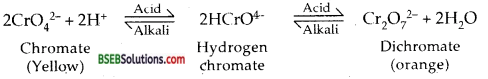Therefore increasing pH, the orange dichromate solution changes to yellow chromate CrO42- solution.

Question 15.
Describe the oxidising action of Potassium Dichromate and write the ionic equations for its reactions with
(a) Iodide,
(b) Iron (II) Solution
(c) H2S.
K2Cr2O7 + 4H2SO4→ K2SO4 + Cr2(SO4)3 + 4H2O + 3[O] or in IONIC FORM. ‘
Cr2O72- + 14H+ + 6e → 2Cr3+ + 7H2O] reduction
∴ Cr2O72- in acid medium is a strong oxidizing agent.
(a) It oxidizes I to I2
Cr2O72-+ 14H+ + 6I →2Cr3+ + 3I2 + 7H2O

(b) It oxidizes Fe (II) to Fe (III) in acidic solution
Cr2O72- + 14H+ + 6Fe2+→2 Cr3+ + 6Fe3+ + 7H2O

(c) It oxidizes H2S to S.
Cr2O72- + 8H+ + 3H2S → 2 Cr3+ + 3s + 7H2O

Question 16.
Describe the preparation of potassium permanganate. How does the acidified permanganate solution reacts with
(a) Iron (II) ions
(b) SO2 and
(c) oxalic acid? Write the ionic equations for the reactions.
Preparation of Potassium permanganate (KMnO4)-It is prepared from pyrolusite ore.
It involves the following steps :
(i) Conversion of pyrolusite ore to potassium manganate-The pyrolusite ore is fused with caustic potash (KOH) or potassium carbonate in the presence of air to give a green mass due to the formation of potassium manganate.(ii) Oxidation of K2MnO4 to KMnO4-The green mass is extracted with water resulting in green solution of potassium manganate. The green solution is then treated with a current of Cl2 or O3 or CO2 to oxidize K2Mn04 to KMnO4.
2 K2MnO4 + Cl2 → 2 KCl + 2 KMnO4
2 K2MnO4 + O3 + H2O → 2 K2MnO4 + 2 KOH + O2.
3 K2MnO4 + 2CO2 → 2 K2CO3 + MnO2 ↑ + 2 KMnO4
The solution is concentrated and dark purple crystals of KMnO4 are obtained.
Alternately, the alkaline K2MnO4 solution is oxidized electrolytically.
Electrolytical oxidation
2K2MnO4 ⇌ 2K+ + MnO42-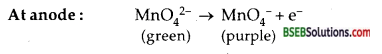At cathode: 2H+ + 2e → H2
Oxidizing action of acidified KMnO4 on
(a) iron (II) ions
2KMnO4 + 3H2SO4 → K2SO4 + 2 MnSO4 + 3H2O+ 5
or in an ionic reaction
MnO4 + 8H+ + 5e → Mn2+ + 4H2O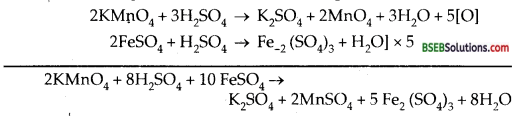or in ionic form
MnO4 + 8H+ + 5Fe2+ → 5 Fe3+ + Mn2+ + 4H2O
(b) SO2or ionic formQuestion 17.
For M2+/M and M3+/M2+ systems the Eθ values for some metals are as follows :
Cr2+/Cr -0.9 Cr3+/Cr2+ -0.4 V
Mn2+/Mn -1.2V Mn3+/Mn2+ +1.5 V
Fe2+/Fe -0.4V Fe3+/Fe2+ +0.8V
Use this data to comment upon:
(a) The stability of Fe3+ in acid solution as compared to that of Cr3+ or Mn3+.
(b) The ease with which iron can be oxidised as compared to a similar process for either chromium or manganese metal.
It is possible to predict from electrode potential data, the stability of different oxidation states in aqueous solutions of transition metal ions.
Fe3+ + e → Fe< sup>2+ + 0.8 V
Cr3+ +e → Cr2+ -0.4 V
Mn3+ + e → Mn2+ + 1.5 V
(a) Cr3+ /Cr2+ has a negative reduction potential. Hence Cr3+ ion cannot be reduced to Cr2+ ion, i.e., Cr3+ ion is most stable. Mn3+/Mn2+ has large positive E° value. Hence Mn3+ can be easily reduced to Mn2+ i.e., Mn3+ is least stable. E° value for Fe3+/Fe2+ is positive but small. Hence Fe3+ is more stable than Mn3+ but less stable than Cr3+.
(b) Oxidation potentials for the given pairs will be + 0.9 V, + 1.2 V and + 0.4 volt. Thus the order of their getting oxidized will be in the order Mn > Cr > Fe.

Question 18.
Predict which of the following will be coloured in aqueous solution?
Ti3+, V3+, Cu+, Sc3+, Mn2+, Fe3+ and CO2+ Give reasons for each.
Leaving aside Cu+, Sc3+ which will be white [ ∵ of 3d10,3d° electronic arrangement], all others will be coloured because they have partially filled d-orbitals and thus will involve d -d transitions.

Question 19.
Compare the stability of + 2 oxidation state for the elements of the first transition series.
With increasing nuclear charge, which accompanies the filling of the innder d orbitals, there is an increase in ionization enthalpy along each series of the transition elements from left to right, but many small variations occur. First three ionization enthalpies of the first row elements shows that although the first ionization enthalpies, in general, increases, the increase in the second and third ionization enthalpies for the successive elements are not of the same magnitude. However, the trend is similar for the second ionization enthalpies, which for the most part increase smoothly as the atomic number increases, the exceptions are chromium and copper for which these values are notably larger than those of their neighbours.

These exceptions are attributed to the extra stability of half-filled or completely filled set of d orbitals in chromium and copper. The third ionization enthalpies are quite high and there is a marked break between the values of Mn2+ and Fe2+. Also, the high values of copper, nickel and zinc indicate why it is difficult to obtain oxidation state greater than two for those elements.
Table: Oxidation states of the first-row transition metals (the most common ones are in coloured bold types)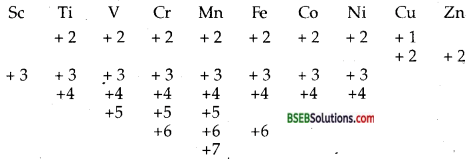[ + 2 oxidation state is common to all transition elements of the first Transition series. ]

Question 20.
Compare the chemistry of actinoids with that of the lanthanoids with special reference to:
(a) electronic configuration,
(b) atomic and ionic sizes,
(c) oxidation state and
(d) chemical reactivity.
(a) Electronic configuration of actinoids and lanthanoids- Both are INNER-TRANSITION ELEMENTS. The inner transition elements or f-BLOCK ELEMENTS are those which have partly filled f- subshells of the antepenultimate orbit in their elementary or ionic state. In both lanthanoids and actinoids the last electron enters (n – 2) f subshell.
Lanthanoids have general electronic configuration of [Xe] 4f0-145d0-16s2
where [Xe] stands for Xe core.
Actinoids have the general electronic configuration of [Rn] 5f0-14 6d0-2 7s2 where [Rn] stands for radon core.
Last electrons enters 4f in the case of lanthanoids and it enters 5f in the case of actinoids.

(b) Atomic sizes of Ln vary from 187 pm for La to 173 for Yb. Their ionic sizes [Ln3+] vary from 106 pm for La3+ to 85 pm for Lu3+, whereas the size of actinoids vary from 111 pm for Ac3+ to 98 pm for Cf3+ and from 99 pm for Th4+ to 86 pm for Cf4+.

(c) Oxidation states-Whereas Lanthanoids show a dominant oxidation state of + 3 [+2, + 4 are exceptions] actinides show variable oxidation state of + 2, + 3, + 4, + 5, + 6, + 7.

(d) The chemical reactivity etc. are summarised below:
Comparison of Lanthanide and Actinide Series-Elements of lanthanide and actinides resemble’ in many respects but they do differ in some respects as shown below :

 Lanthanides Actinides Similarities1. Mainly exhibit + 3 oxidation state. 1. They also mainly exhibit + 3 oxidation state. 2. They exhibit lanthanide contraction. 2. They exhibit actinide contraction. 3. They show ion-exchange behaviour. 3. They also show ion-exchange behaviour.
 Lanthanides Actinides Differences1. In addition to + 3 oxidation state, they exhibit + 2 and + 4 oxidation states only. Differences1. In addition to + 3 oxidation state, they show +4, +5, +6 and +7 oxidation states. 2. Most of their ions are colourless. 2. Most of their ions are coloured. 3. They do not form complexes easily. 3. They have much greater tendency form complexs. 4. They do not form oxoca lions. 4. They form oxidations SuCh UO22+, PuO22+ and UO+. 5. Their compounds are less basic. 5. Their compounds are more basic. 6. Except promethium. they are non-radioactive. 6. They are radioactive. 7. Their magnetic properties can be easily explained. 7. Their magnetic properties cannot be easily explained.

Question 21.
How would you account for the following:
(a) Of the d4 species, Cr2+ is strongly reducing while manganese (III) is strongly oxidising.
(b) Cobalt (II) is stable in aqueous solution but in the presence of complexing reagents, it is easily oxidised.
(c) The d1 configuration is very unstable in ions.
(a) Of the d4 species Cr2+ is strongly reducing while Mn (III) is strongly oxidizing.
(i) Cr2+ + 2e→ Cr E°red = – 0.90 V
(ii) Cr → Cr2+ + 2e E°OX = + 0.90
The chances of (ii) occurring are more as oxidation potential of the reaction is + ve and change from d4 to d3 which is more stable [E32g ].
Mn3+ + e → Mn2+ E°red = +1.57 V
∴ Mn3+ can readily change to Mn2+ [its reduction potential is positive]
∴ Mn3+ is strongly oxidizing, as it involves change from 3d4 to 3d5 which is more stable configuration.

(b) CO2+ + 2e → CO -0.28 V
Cobalt (II) is quite stable in aqueous solution as its reduction potential is – ve. Co (III) has greater tendency to form coordination complexes than Co (II), hence in the presence of complexing agents or ligands.
CO2+ → CO3+ + e + 1.97 V
Co2+ can be easily oxidized to Co3+, due to CFSE which more than compensates the 3rd IE.

(c) Except vanadium (IV), all other with d1 configuration are either reducing or undergo disproportionation. For example, disproportionation occurs for Cr (V) and Mn (VI) as follows:
3CrO43- + 8H+ → 2CrO42- + Cr3+ + 4H2O
3MnO42- + 4H+ → 2 MnO4 + MuO2 + 2H2O
The hydration or lattice energy more than compensates the IE involved in removing electron from d1.

Question 22.
What is meant by disproportionation? Give two examples of disproportionation reaction in aqueous solutions.
Disproportionation reactions are those in which an element undergoes as increase as well as a decrease in its oxidation state i.e., it gets oxidized as well as reduced in the same reaction e.g.(i) In the (i) above Cr having an oxidation state of + 5 in CrO43- changes its ox. state to + 6 in CrO42- and + 3 in Cr+3.
(ii) In the (ii) above Mn (ox. no. + 6 in MnO42-) changes its ox. state to +7 in MnO4 and to + 4 in MnO2.

Question 23.
Which metal in the first series of transition metals exhibits +1 oxidation state most frequently and why?
Cu which has the electronic configuration of 3d104s1 is the metal which exhibits the + 1 oxidation state most frequently after the removal of 1 electron from 4s1, inner d subshell is fully filled, i.e., 3d10.

Question 24.
Calculate the number of unpaired electrons in the following gaseous ions: Mn3+, Cr3+, V3+ and Ti3+ which one of these is the most stable in aqueous solution,
Mn3+ = 3d4; 4 unpaired electrons
Cr4+ = 3d3; 3 unpaired electrons
V3+ = 3d2; 2 unpaired electrons
Ti3+ = 3dM1; 1 unpaired electrons
Cr3+ is most stable in aqueous solutions out to all of them.

Question 25.
Give example and suggest reasons for the following features of the transition metal chemistry :
(a) The lowest oxide of transition metal is basic, the highest is amphoteric/acidic,
(b) A transition metal exhibits highest oxidation state in oxides and fluorides,
(c) the highest oxidation state is exhibited in oxoanions of a metal.
(a) In general, the oxides in the lower oxidation state are basic and in their higher oxidation states, they are acidic. For example MnO [+ 2] is basic and Mn2O7 is acidic. MnO dissolves in acids like HCl, so it is basic. Mn2O7, CrO3 (higher oxidation state) forms acids like HMnO4 and H2CrO4.

(b) A transition metal exhibits higher oxidation states in oxides and fluorides as fluorine and oxygen are highly electronegative elements (and strongest oxidizing agents). For example Os shows an ox. state of + 6 in OsF6 and V shows an ox. state of + 5 in V2O5.
(c) Oxometal anions have highest oxidation state, e.g., Cr in Cr2O72- has an oxidation state of + 6 whereas Mn in MnO42- has an oxidation state of + 7. This is again due to the combination of the metal with oxygen, which is highly electronegative and oxidizing element.

Question 26.
Indicate the steps in the preparation of:
(a) K2Cr2O7 from chromite ore
(b) KMnO4 from pyrolusite ore
(a) Potassium Dichromate (K2Cr2O) is prepared from chromite ore [FeCr2O4]. The various steps involved are:
1. Preparation of sodium chromate-The powdered ore is heated with molten alkali in the presence of air in a reverberatory furnace to produce sodium chromate.
4 FeCr2O4 +16 NaOH + 7O2→ 8Na2CrO4 + 2Fe2O3 + 8H2O

2. Conversion of sodium chromate into sodium dichromate. Sodium chromate is extracted with water and acidified with sulphuric acid to get sodium dichromate.
2Na2CrO4 + H2SO4 → Na2Cr2O7 + Na2SO4 + H2O
On cooling, sodium sulphate separates out as Na2SO4. 10H2O and the solution contains sodium dichromate in it.

3. Conversion of sodium dichromate into potassiutn dichromate. The solution containing sodium dichromate is treated with potassium chloride, (calculated amount)
Na2Cr2O7 + 2KCl → K2Cr2O7 + 2NaCl
K2Cr2O7 being least soluble separates out and is removed by filtration. On drying, orange crystals of K2Cr2O7 are obtained.
The dichromate ion Cr2O72- and chromate ion CrO42- exist in equilibrium with each other at a pH of about 4. They are interconvertible, by changing the pH of the solution.Therefore increasing pH, the orange dichromate solution changes to yellow chromate CrO42- solution.

(b) Preparation of Potassium permanganate (KMnO4)-It is prepared from pyrolusite ore.
It involves the following steps :
(i) Conversion of pyrolusite ore to potassium manganate-The pyrolusite ore is fused with caustic potash (KOH) or potassium carbonate in the presence of air to give a green mass due to the formation of potassium manganate.(ii) Oxidation of K2MnO4 to KMnO4-The green mass is extracted with water resulting in green solution of potassium manganate. The green solution is then treated with a current of Cl2 or O3 or CO2 to oxidize K2Mn04 to KMnO4.
2 K2MnO4 + Cl2 → 2 KCl + 2 KMnO4
2 K2MnO4 + O3 + H2O → 2 K2MnO4 + 2 KOH + O2.
3 K2MnO4 + 2CO2 → 2 K2CO3 + MnO2 ↑ + 2 KMnO4
The solution is concentrated and dark purple crystals of KMnO4 are obtained.
Alternately, the alkaline K2MnO4 solution is oxidized electrolytically.
Electrolytical oxidation
2K2MnO4 ⇌ 2K+ + MnO42-At cathode: 2H+ + 2e → H2
Oxidizing action of acidified KMnO4 on
(a) iron (II) ions
2KMnO4 + 3H2SO4 → K2SO4 + 2 MnSO4 + 3H2O+ 5
or in an ionic reaction
MnO4 + 8H+ + 5e → Mn2+ + 4H2Oor in ionic form
MnO4 + 8H+ + 5Fe2+ → 5 Fe3+ + Mn2+ + 4H2O
(b) SO2or ionic formQuestion 27.
What are alloys? Name an important alloy which contains some of the lanthanoid metals. Mention its uses.
An alloy is a homogeneous mixture of two or more metals or metals and non-metals. An important alloy containing lanthanoid 1 metals is MISCH metal which contains 95% lanthanoid metal and iron is 5% along with traces of S, C, Ca and Al. It is used in Mg-based alloy to produce bullets, shells and lighter flints. (2) Magnesium mixed with 3% mischmetal (to increase its strength) is used in making jet engine parts.

Question 28.
What are inner transition elements? Decide which of the following atomic numbers are the atomic number of the inner transition elements : 29,59,74,95,102,104.
The f-block elements, i.e., in which the lost electron enters into f-subshell of the antepenult bate orbit are called inner-transition elements. They include lanthanoids (58-71) in which the last electron ‘ enters 4f subshell and actinoids (90 – 103) in which the last electron enters 5f subshell. The elements with atomic numbers 59,95 and 102 are inner transition elements.

Question 29.
The chemistry of the actinoids elements is not so smooth as that of the lanthanoids. Justify this statement by giving some examples from the oxidation state of these elements.
The chemistry of the actinoids is highly complicated unlike the chemistry of the lanthanoids. The chemistry of the lanthanoids is very similar whereas actinoids differ vastly in their chemical behaviour. Take, for instance, the oxidation states. Lanthanoids show a remarkably stable oxidation state of + 3, with a few exceptions of + 2 and + 4, whereas actinoids show several oxidation states of not only + 3, but + 2, + 4, +- 5, + 6, + 7. Neptunium (Z = 94) shows oxidation states of + 3, + 4, + 5 and + 7 etc. This is due to small energy difference between 5f, 6d and 7s subshells of the actinoids.

Question 30.
Which is the last element in the series of actinoids? Write the electronic configuration of this element. Comment on the possible oxidation state of this element.
Lawrencium (Lr) in the series of actinoids is the last element. Electronic configuration = [Rn]86 5f14 6d1 7s2 Possible oxidation state + 3, after the removal of one electron from 6d and two from 7s. [ ∵ 5f14 is most stable].

Question 31.
Use Hund’s rule to derive the electronic configuration of Ce3+ ion and calculate its magnetic moment on the basis of “spin-only formula”.
Ce+3 = [Xe]4f1; Ce3+ is paramagnetic
µmagnetic = 𝑛(𝑛+2)‾‾‾‾‾‾‾‾√
n = number of unparied electrons
Ce3+ has only one unpaired electron of 4f’ subshell
i.e. n = 1 .
∵µmagnetic = 1(1+2)‾‾‾‾‾‾‾‾√ = 3‾√ = 1.73 B.M.

Question 32.
Name the members of the lanthanoid series which exhibit + 4 oxidation states and those which exhibit + 2 oxidation states. Try to correlate this type of behaviour with the electronic configurations of these elements.
The elements Pr, Ce, Tb, Dy show oxidation states of + 4 in Pr4t, Ce4+, Tb4+, Dy4+. The elements showing an oxidation state of + 2 are Sm (Samarium), Eu (Europium), Tm (Thullium), Yb (Ytterbium) in Sm2+, Eu2+,Tm2+, Yb2+.
The oxidation states such as + 2 or + 4 exhibited by the lanthanides can be explained on the basis of extra-stability associated with empty, half-filled and completely filled 4f subshell. For example, Eu, Yb exhibit + 2 oxidation state because they acquire the f and f14 configuration respectively. Similary Ce, Tb show + 4 oxidation state by acquiring f° and f7 configurations respectively.

Question 33.
Compare the chemistry of the actinoids with that of lanthanoids with reference to
(i) electronic configuration,
(ii) oxidation states,
(iii) chemical reactivity.

Question 34.
Write the electronic configuration of the element with the atomic numbers 61,91,101 and 109.
Promethium Pm = 61 = [Xe] 4f56s2
Protactinium = Pa = 91 = [Rn] 5f2 6d1 7s2
Mendelevium = Md = 101 = [Rn] 5f13 7s2
Meitnerium = Mt = 109 = [Rn]5f14 6d7 7s2.

Question 35.
Compare the general characteristics of the first series of the transition metals with those of the second and third series metals in the respective vertical columns. Give special emphasis on the following points:
(i) electronic configurations,
(ii) oxidation states,
(iii) ionisation enthalpies and
(iv) atomic sizes.
(i) Electronic Configuration-The general electronic configuration of the first transition metals from Sc (21) to Zn (30) are [Ar] 3d14s2 to [Ar] 3.d10 4s2 respectively. The electronic configurations in the valence shells of the 2nd and 3rd transition series are similar with a few exceptions as depicted below. In the case of 3rd transition series, after La (57), 4f subshell is filled (4f14) for elements from Hf (72) to Hg (80).(ii) Generally shown oxidation states of transition metals (Very rare oxidation states are given in parenthesis)The transition elements have their valence electrons in two different sets of orbitals, i.e., (n – 1) d and ns. Since there is very little difference in the energies of these orbitals, both energy tends can be used for bond formation. If two electrons from s-orbital are used for bond formation, it gives an oxidation state of + 2 to the transition metals.
If (n – 1) d orbital electrons are also used in addition to the ns orbital electrons, it gives variable oxidation states to the transition elements. The most common oxidation state of I transition series is + 2 except Sc (+3). It follows from this that d-orbitals are more stable than the s-orbital after Sc.
Mostly ionic bonds are formed in + 2 and + 3 oxidation states. Higher oxidation states result from covalent bonding, e.g., all the bonds between Mn and Q in MnO4 are covalent.

The maximum oxidation state increases with atomic number within a group. For example the common oxidation states for iron are + 2 and + 3, whereas ruthenium (Ru) and osmium (Os) of the same group show the oxidation states of + 4, + 6, + 8. Highest oxidation shown by any transition element is + 8.
Transition elements also show low oxidation states of + 1 and zero in their compounds. For example nickel in Ni(CO)4 is in zero oxidation state.

(iii) Ionisation Energies-The first ionisation energies of d-block elements lie between s-block and p-block elements. They are higher than those of s-block elements and are lesser than those of p-block elements.
(a) The ionisation energy gradually increases across a given transition series with increase in atomic number.
In a given series, the difference in the ionisation energies between any two successive d-block elements is very much less than the difference in case of successive s-block or p-block elements.

(b) The first ionisation energies of Zn, Cd and Hg however, very high because of the fully filled (n – 1) d10 ns2 configuration. The high values of 3rd ionisation energies for Cu, Ni and Zn explain why they have a maximum oxidation state of + 2.

(c) The first ionisation energies of 5d elements [3rd Transition series] are higher as compared to those of 3d and 4d elements. This is because the weak shielding of nucleus by 4f electrons in 5d elements results in greater effective nuclear charge acting on the outer valence electrons. The ionisation energies of I and II transition metals are irregular.

(iv) The atomic radii increase down the group. This means that the atomic radii of second series are larger than those of the first transition series. But the atomic radii of the 2nd and 3rd transition series are almost the same. It is due to lanthanide contraction.

Question 36.
Write down the number of 3d electrons in each of the following ions: Ti2+, V2+, Cr3+, Mn2+, Fe2+, Fe3+, CO2+, Ni2+ and Cu2+. Indicate how would you expect the five 3d orbitals to be occupied for these hydrated ions (octahedral).
(a) Ti2+ : 3d2: It has two electrons in 3d orbitals.
V2+ : 3d3: It has three electrons in 3d orbitals.
Cr3+: 3d3: It has three electrons in 3d orbitals
Mn2+ : 3d5: It has five electrons in 3d orbitals.
Fe2+ : 3d6: It has six electrons in 3d orbitals.
Fe3+ : 3d5: It has five electrons in 3d orbitals.
Co2+: 3d7: It has seven electrons in 3d orbitals.
Ni2+ : 3d8: It has eight electrons in 3d orbitals.
Cu2+ : 3d9 : ft has nine electrons in 3d orbitals.

(b) [Ti (H2O)6]2+: Two out of 3d orbitals will have one unpaired electron in each of them and three will have two electrons each denoted . by HzO ligands.
[V (H2O)6]2+: It will have 3 unpaired electrons in three out of five 3c1 orbitals and remaining two out of 3d orbitals will have two electrons each donated by H2O,
[Cr(H2O5)6]3+: It has three unpaired electrons in three out of five 3d orbitals and remaining two fully filled by 11,0 ligands.
[Mn (H2O)6]2+: It will have four out of the five 3d orbitals fully filled [ 2 electrons in each] two from H20 ligands and one will have one unpaired electron.
[Fe (H2O)6]2+: It will have all the five 3d orbitals fully filled [2 electrons in each orbital] – last two will have electrons donated by H2O ligands.
[Fe (H2O)6]3+: All the five 3d orbitals will have one unpaired electron in each one of them.
[Co(H2O)6]2+:4 out of five 3d orbitals will be fully filled and one will have one unpaired electron.
[Ni (H2O)6]2+: It will have first three of five 3d orbitals occupied by two electrons each and the remaining two having one electron each.
(Cu (H2O)6]2+: It will have four out of five 3d orbitals fully paired and are having one electron.

Question 37.
Comment on the statement that elements of the first transition series possess many properties different from those of heavier transition elements.
Elements of the first transition series differ in many properties from those of heavier transition elements particularly of the 3rd transition series because of the presence of 4f orbitals in them and from the elements of the 4th incomplete transition series because of the presence of 5f orbitals in them.

Question 38.
What can be inferred from the magnetic moment value of the following complex species? ‘

 Example Magnetic Moment (BM) K4[Mn(CN)6] 2.2 [Fe (H2O)6]2+ 5.3 [K2(MnCl4) 5.9

Magnetic moments determined by the number of unpaired electrons and is calculated by spin only formula, i.e.,
µ = 𝑛(𝑛+2)‾‾‾‾‾‾‾‾√
where n is the number of unpaired electrons and µ is magnetic moment in units of Bohr magneton (BM). A single unpaired electron has a magnetic movement of 1.73 Bohr Magnetons (BM).
Magnetic movement increases with the increasing number of unpaired electrons, e.g.

(a) K4[Mn(CN)6] µ = 𝑛(𝑛+2)‾‾‾‾‾‾‾‾√
Configuration 3d5 or BM = 2.2
unpaired electrons 5
Hybridisation – d2 sp3 and CN is a strong legand

(b) [Fe(H2O)6]2+
Configuration Fe – 3d6 µ = 𝑛(𝑛+2)‾‾‾‾‾‾‾‾√
unpaired electrons 4
Calculated BM = 4.90 BM 5.3 to 5.5
Hybridisation sp3d2 and H2O is a weak ligand

(c) [K2(Mn Cl4)]
Configuration = 3d5 µ = 𝑛(𝑛+2)‾‾‾‾‾‾‾‾√
unpaired electrons = 5
Calculated BM = 5.9 p = 5.9 BM
Hybridisation sp3 and Cl is a weak ligand.

### Bihar Board Class 12 Chemistry The d-and f-Block Elements Additional Important Questions and Answers

Question 1.
Why are transition elements known as d-block elements? (P.S.B/03)
Because the last electron enters (n – 1) d-orbital, i.e., d-orbital of the penultimate shell.

Question 2.
Why does a transition series contain 10 elements? (P.S.B. 2000/01)
There are five d-orbitals in an energy level and each orbital can have two electrons. Thus we have 10 elements in a transition series.

Question 3.
Write the general electronic configuration of transition elements or d-block elements. (A.I.S.B. 99, P.S.B. 99,2000)
(n – 1) d1-10 ns0-2.

Question 4.
Why lanthanides are called f-block elements? (HPSB 96, HSB 99)
This is because the last electron in them enters into f-orbital.

Question 5.
Give the general electronic configuration of actinoids. (P.S.B. 97)
5f1-14 6d0-1 7s2.

Question 6.
Why Cd2+ salts are white? (H.P.S.B.’ 97, H.S.B. 2001, P.S.B. 2003)
It has completely filled d-orbitals (d10).

Question 7.
Write ionic equation for the reaction between Cr2O72- ions and Fe2+ ions in acidic medium.
Cr2O72- + 14H+ + 6 Fe2+→2Cr3+ + 6 Fe3+ + 7H2O.

Question 8.
Write ionic reaction between MnO4 ions and oxalate ions at 333 K. (P.S.B. 2005)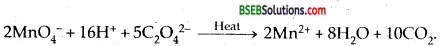Question 9.
Which block of elements in the periodic table forms complexes readily? (H.D.S.B. 2004)
d-Block.

Question 10.
What are coinage metals?
Cu, Ag and Au metals, which have been used in making of coins.

Question 11.
What is the effect of increasing PH on K2Cr2O7 solution? (A.I.S.B. 2003)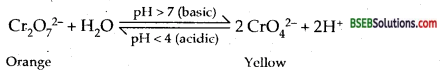Question 12.
Which divalent metal ion has maximum paramagnetic character among the first transition metals? Why? (H.P.S.B. 2002)
Mn2+ because of presence of maximum no. of unpaired electrons i.e., 5.

Question 13.
Name a transition metal which does not exhibit variation in oxidation state in its compounds. (A.I.S.B. 2002)
Zinc (Zn).

Question 14.
Name any three elements of second transition series. (H.P.S.B. 2002)
Cadmium (Cd), Silver (Ag), Zirconium (Zr).

Question 15.
Write the formula of a compound where transition metal is in + 7 oxidation state. (A.I.S.B. 1999, H.P.S.B. 2002)
KMnO4.

Question 16.
Why does Vanadium pentoxide act as a catalyst?
Vanadium exists in multiple oxidation states.

Question 17.
Which is the most common oxidation state of lanthanides? (A.I.S.B/ 96, H.S.B. 2001, P.S.B. 2001, 2003)
+ 3.

Question 18.
Why is copper (At. No. 29) considered a transition metal?
In its + 2 oxidation state (Cu2+) it has incompletely filled 3d subshell (3d9).

Question 19.
The size of the divalent cations in the lanthanide series decreases steadily as the atomic number increases. What is this known as?
Lanthanide Contraction.

Question 20.
Why in permanganate ion, there is a covalency between Mn and Oxygen? (P.S.B. 2005)
In MnO4 ion, Mn is in highest oxidation state, viz, + 7. In highest oxidation transition metals form covalent bonds.

Question 21.
Write all possible oxidation states of an element having atomic number 25. (A.I.S.B. 2005)
Elecbonic configuration of 25Mn = [Ar]18 3d54s2. It shows oxidation states of + 2, + 3, + 4, + 5, + 6 and + 7.

Question 22.
Evaluate the magnetic moment of a divalent ion in aqueous solution if its atomic number is 25. (N.C.E.R.T.)
Z = 25 for Mn. Mn = [Ar]18 3d54s2.
∴ Mn2+ = [ Ar]18 3d5. It has 5 unpaired elecbons.
Magnetic moment µ = 𝑛(𝑛+2)‾‾‾‾‾‾‾‾√ = 5(5+2)‾‾‾‾‾‾‾‾√ =5.92B.M.

Question 23.
On what ground can you say that Scandium (Z = 21) is a transition element, but zinc (Z = 30) is not?
Sc = [Ar]18 3s14s2 has partially filled (n-1) d orbitals. Hence it is a transition element. Zn = 3d10 4s2 has completely filled (n – 1) d orbital (3d10) in its atomic as well as ionic state.

Question 24.
Why is the third ionisation energy of manganese [At No = 25] unexpectedly high?
Mn2+ = [Ar]18 3d5 has half-filled orbitals and is quite stable. Hence it is difficult to remove the 3rd electron.

Question 25.
Explain why lanthanides are paramagnetic in nature?
All lanthanides except La3+ and Lu3+ contain unpaired electrons.

Question 26.
Why is the separation of lanthanides difficult?
Due to lanthanides contraction, there is very small change in their atomic and ionic radii. Thus similarity in chemical properties of lanthanides makes their separation difficult.

Question 27.
Why are the transition elements so named?
Because their properties are between those of s-block and p- block elements.

Question 28.
Name the elements which do not really transition elements but are discussed with them.
Zinc (Zn), Cadmium (Cd) and mercury (Hg).

Question 29.
Though copper and gold have completely filled d-orbitals, yet are considered as transition elements. Why?
Because their cations have only partially filled sets of d- orbitals.

Question 30.
Copper (I) compounds are white while copper (II) compounds are coloured. Why?
Copper (I) has all d-orbitals filled (3d10) whereas copper (II) has partially filled d-orbitals (3d9).

Question 1.
Why Sm2+, Eu2+ and Yb2+ ions in solutions are good reducing agents, but an aqueous solution of Ce4+ is a good oxidizing agent. (P.S.B. 2003)
The most stable oxidation state of Lanthanides is + 3. Hence ions in + 2 state tend to change to + 3 state by loss of electron and those in + 4 state tend to gain electrons to get into + 3 state.

Question 2.
Ce (III) among the lanthanides can be esaily oxidized to Ce (IV). Explain why? (D.S.B. 97), (A.I.S.B. 2002)
Ce (III) having the configuration 4f1 5d° 6s° can easily lose electron to acquire the configuration 4f° and form Ce (IV).

Question 3.
The + 3 oxidation states of Lanthanum (Z = 57), gadolinium (Z = 64) and lutetium (Z = 71) are especially stable. Why?
This is because they have empty, half-filled and completely filled 4f subshell respectively (4f°, 4f7 and 4f14).

Question 4.
La(OH)3 is more basic than Lu(OH)3 Explain.
As the size of Lanthanide ions decreases from La3+ to Lu3+, the covalent character of the hydroxides increases [FAZAN’S Rule]. Hence the basic strength decreases from La (OH)3 to Lu (OH)3.

Question 5.
Explain how the colour of K2Cr2O7 solution depends upon the PH of the solution. (Haryana B. 1997)
In the solution the following equilibrium exists:
Cr2O72-+H2O ⇌ 2CrO42- + 2H+
In acidic medium (PH < 7) it exists as Cr2O72- ions and hence has orange colour. In-basic medium (PH > 7) it exists as CrO42- ions and therefore is yellow in colour.

Question 6.
K2Pt Cl6 is known but corresponding Ni compound is not known state reason for it. (A.I.S.E. 1993)
This is because Pt4+ is more stable than Ni4+ as the sum of 4IE’s of Pt is less than that of Ni.

Question 7.
How many water molecules are in involved in coordination in CuSO4.5H2O?
Four water molecules are coordinated to Cu2+ ion and fifth is attached to anion by hydrogen bonding.

Question 8.
(a) Write a metal oxide compound for manganese in each of the following oxidation states: +2, + 3, + 4, + 6, + 7.
(b) List these metal oxides in the decreasing acidic character.
(a) MnO, Mn2O3, MnO2, MnO3, Mn2O7.
(b) Decreasing order of acidic character.
Mn2O7> MnO3 > MnO2 >Mn2O3> MnO.

Question 9.
Why are the IEs of 5d elements greater than 3d elements?
In the 5d series/after La (57), there is lanthanide contraction. As a result, in each group the atomic size of 5d element is small and its nuclear charge is large. Hence the ionisation energies of 5d elements are larger than 3d elements.

Question 10.
A compound has been found to have magnetic moment of 3.9 B.M. How many unpaired electrons does it contain?
µ = 𝑛(𝑛+2)‾‾‾‾‾‾‾‾√ B.M.
∴3.9 = 𝑛(𝑛+2)‾‾‾‾‾‾‾‾√
Solving for n; n = 3
It contains 3 unpaired electrons.

Question 11.
Transition elements exihibit higher enthalpies of atomisation. Explain why?
Enthalpy of atomisation is the amount of heat required to break the metal lattice to get free atoms. As transition metals contain a large number of unpaired electrons, they have strong interatomic attractions (metallic bonds). Hence, they have high enthalpies of atomisation.

Question 12.
The melting and boiling points of Zn, Cd, and Hg are low. Why?
In Zn, Cd, and Hg all the electrons in d-subshell are paired. Hence the metallic bonds present in them are weak. That is why they have low melting and boiling points.

Question 13.
Explain why transition elements have many irregularities in their electronic configuration? {P.S.B. 2001,2003)
In the transition elements, the (n – 1) d subshell and ns subshell have very small in energies. The incoming electron may enter
into ns or (n – 1) d subshell. Hence they show irregularities in their electronic configurations.

Question 14.
Chromium is a typical hard metal while mercury is a liquid. Why?
Chromium (Cr) has five unpaired d electrons in the d subshell (3d54S1). Hence metallic bonds are strong. In mercury, all the d-orbitals are fully filled (3d10 4s2). Hence the metallic bonding is weak.

Question 15.
Why the properties of third transition series are very similar to second transition series? (P.S.B. 2001, H.S.B. 2002, D.S.B. 91)
In the 3rd transition series, after lanthanum, there is lanthanide contraction. Due to this contraction, the size of any atom of the third series is almost the same as that of the element lying just above it in the second transition series. This leads to similarity in their properties.

Question 16.
Among the ionic species Sc3+, Ce4+ and Eu2+ which one is a good oxidizing agent? Give a suitable reason for your answer. [Atomic No. Sc = 21, Ce = 58, Eu = 63).
Sc3+ is having the stable electronic configuration of the noble gas [Ar]. Therefore it has no tendency to acquire an electron. The typical (stable) oxidation state of lanthanides is + 3. Ions with oxidation state + 2 or + 4 tend to change to + 3 by loss or gain of electrons. Ce4+ gains electron to change to Ce3+. Hence it is a good oxidizing agent.

Question 17.
The chemistry of the actinoids is not so smooth as that of lanthanoids. Justify this statement by giving some examples from the oxidation state of these elements.
Ans:
Lanthanoids show limited number of oxidation states viz., + 2, -r 3 and + 4 (out of which + 3 is most common). This is because of large energy g;ap between 4f, 5d and 6s subshells. The dominant oxidation state of actinoids is also + 3, but they show a number of other oxidation states also, e^g., Uranium (Z = 92) and Plutonium (Zn = 94) show + 3, + 4, + 5 and + 6, neptunium (Z = 94) shows -KT, + 4, + 5 and a- 7 etc. This is due to small energy difference between 5f, 6d and 7s subshells of the actinoids.

Question 18.
The 4d and 5d series of transition metals have more frequent metal-metal bonding in their compounds than do the 3d metals. Explain. (D.S.B. 2004)
In the same group of d-block elements, the 4d and 5d transition element has larger size than that of 3d element. Thus, the valence electrons are less tightly held and hence can form metal-metal bond more frequently.

Question 19.
[Ti (H2O)6]3+ is coloured while [Sc (H2O)6]3+ is colourless. Explain. (P.S.B. 96,01, H.S.B. 2002)
Ti3+has one in the 3d orbital which can absorb energy corresponding to yellow wavelength and jump from t2g to eg set of d- orbitals. But Sc3+ has no electron in the 3d orbital.

Question 20.
Why are the ionisation energies of 5d elements greater than 3d elements? (A.I.S.B. 91, H.R.S.B. 98, H.S.B. 96,2003,2004, J & K 1996)
In the 5d series, after lanthanum (Z = 57), there is lanthanide contraction. In each group, the size of the 5d element is smaller while nuclear charge is greater than 3d element. Hence ionisation energies of 5d elements are greater than 3d elements.

Question 1.
Explain the following properties of transition elements of 3d series.
(i) Complex formation
(ii) Catalytical behaviour
(iii) Magnetic character,
(iv) Colour.
(i) Formation of Complex Compounds-Complex compounds are those in which the metal ions bind a number of anions or neutral molecules giving complex species with characteristic properties. A few examples are: [Fe(CN)6]3-, [Fe(CN)6]4-, [Cu(NH3)4]2+ and [PtCl4]2- The transition metals form a large number of complex compounds. This is due to the comparatively smaller sizes of the metal ions, their high ionic charges and the availability of d orbitals for bond formation.

(ii) Catalytical behaviour-The transition metals and their compounds are known for their catalytic activity This activity is ascribed to their ability To adopt multiple oxidation states and to form complexes. Vanadium(V) oxide (in Contact Process), finely divided iron (in Haber’s Process), and nickel (in Catalytic Hydrogenation) are some of the examples. Catalysts at a solid surface involve the formation of bonds between reactant molecules and atoms of the surface of the catalyst (first-row transition metals utilise 3d and 4s electrons for bonding).

This has the effect of increasing the concentration of the reactants at the catalyst surface and also weakening of the bonds in the reacting molecules (the activation energy is lowered). Also because the transition metal ions can change their oxidation states, they become more effective ‘ as catalysts. For example, iron(III) catalyses the reaction between iodide and persulphate ions,
2 I + S2O82-→ I2 + 2 SO42-
An explanation of this catalytic action can be given as:
2 Fe3+ + 2I → 2 Fe2+ + I2
2 Fe2+ + S2O82-→ 2 Fe3+ + 2SO42-

(iii) Magnetic character-Most of the transition metal ions are paramagnetic, i.e., they are attracted by a magnetic field. Paramagnetism arises from the presence of impaired electrons each such electron having a magnetic moment associated with its spin angular momentum and orbital angular momentum. For the compounds of the first transition series, the contribution of the orbital angular momentum is effectively quenched and hence is of no. significance. For these the magnetic moment is determined by the number of unpaired electrons and is calculated by using the ‘spin only formula, i.e., µ = 𝑛(𝑛+2)‾‾‾‾‾‾‾‾√ B.M. or µb, where n = no. of unpaired electrons and µ is magnetic moment in Bohr Magneton (µg).

A single Is electron has a magnetic moment of 1.73 B.M. (µB). The magnetic moment increases with the increasing number of unpaired electrons. Thus, the observed magnetic moment gives a useful indication about the number of unpaired electrons present in the atom, molecule or ion.

(iv) Colour of 3d-series metal ions-When an electron from a lower energy d orbital is excited to a higher energy d-orbital (d-d interactions), it absorbs energy corresponding to a particular wavelength of visible light. The wavelength of the transmitted light belongs to the complementary colour. The frequency of the light absorbed is determined by the nature of the ligand. All those ions having completely filled 3d orbitals (3d10) or completely empty 3d-orbitals (3d°) are colourless whereas those ions with incompleted-orbitals (3d1-9) show different colour as shown below: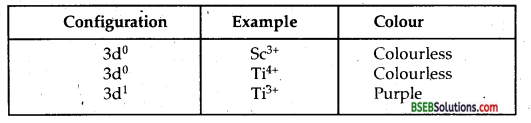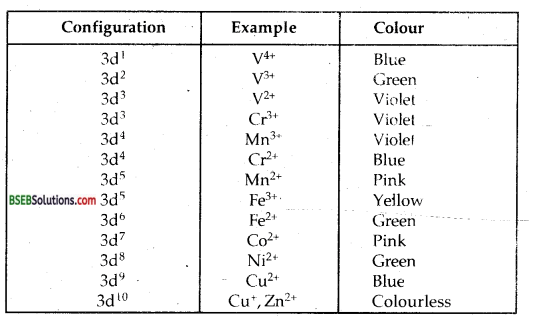Question 2.
What is Lanthanoid contraction? What is its cause? What are the effects of Lanthanoid contraction? Do actinoids also show actinoid contraction similar to lanthanoid contraction?
Lanthanoid contraction-There is overall decrease in atomic and ionic radii from lanthanum to lutetium (La to Lu). It is called lanthanide or lanthanoid contraction. As we proceed from La (57) to Lu (71), there is a regular decrease in their ionic radii in + 3 oxidation state from 106 pm to 86 pm [On average 1.5 pm per Ln3+ ion]. This cumulative effect of the contraction of the lanthanoid series is called lanthanoid contraction.

Cause of lanthanoid contraction-Cause of lanthanoid contraction is due to the imperfect shielding of one 4f electron by another 4f electron with the increase in nuclear charge because of the highly diffused shape of f-orbitals.

Effects of Lanthanoid Contraction-Lanthanoid contraction has far-reaching consequences in the chemistry of the third transition series of the elements as described :
(i) Difficulty in Separation of lanthanoids-Since the change in ionic radii (size of the ions) in Lanthanoids is very small, their chemical properties are similar. This makes the separation of the elements in the pure state difficult. The small difference in their sizes results in the differences in properties like solubility, complex ion formation, hydration, basic character of their hydroxides. These differences enable these elements separation from each other by ion-exchange methods.

(ii) Similarity in size of elements belonging to same group of second and third transition series.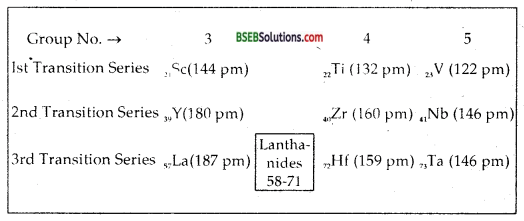The similarity in size of the atoms of the elements belonging to the same group of the 2nd and 3rd transition series (after lanthanum) (e.g. rZr ≈ rHf and rNb = rTa etc.) is evidently due to the lanthanide contraction. Due to similar sizes Zr and 4f exist together in nature and it leads to difficulty faced in their separation.

(iii) Effect on the basic strength of hydroxides-As the size of lanthanoid ions decreases from La3+ to Lu3+, the covalent character of the hydroxides increases and hence the basic strength decreases. Thus La (OH)3 is most basic whereas Lu (OH)3 is least basic. Actinoids show actinoid contraction like lanthanoid contraction-The actinides/actinoids show actinide contraction (very much similar to lanthanoid contraction) due to poor shielding effect of the 5f-electrons. As a result, the radii of the atoms or ions of these metals decrease regularly across the series.

## Bihar Board Class 12th Chemistry The d-and f-Block Elements Textbooks for Exam Preparations

Bihar Board Class 12th Chemistry The d-and f-Block Elements Textbook Solutions can be of great help in your Bihar Board Class 12th Chemistry The d-and f-Block Elements exam preparation. The BSEB STD 12th Chemistry The d-and f-Block Elements Textbooks study material, used with the English medium textbooks, can help you complete the entire Class 12th Chemistry The d-and f-Block Elements Books State Board syllabus with maximum efficiency.

## FAQs Regarding Bihar Board Class 12th Chemistry The d-and f-Block Elements Textbook Solutions

#### Can we get a Bihar Board Book PDF for all Classes?

Yes you can get Bihar Board Text Book PDF for all classes using the links provided in the above article.

## Important Terms

Bihar Board Class 12th Chemistry The d-and f-Block Elements, BSEB Class 12th Chemistry The d-and f-Block Elements Textbooks, Bihar Board Class 12th Chemistry The d-and f-Block Elements, Bihar Board Class 12th Chemistry The d-and f-Block Elements Textbook solutions, BSEB Class 12th Chemistry The d-and f-Block Elements Textbooks Solutions, Bihar Board STD 12th Chemistry The d-and f-Block Elements, BSEB STD 12th Chemistry The d-and f-Block Elements Textbooks, Bihar Board STD 12th Chemistry The d-and f-Block Elements, Bihar Board STD 12th Chemistry The d-and f-Block Elements Textbook solutions, BSEB STD 12th Chemistry The d-and f-Block Elements Textbooks Solutions,
Share: